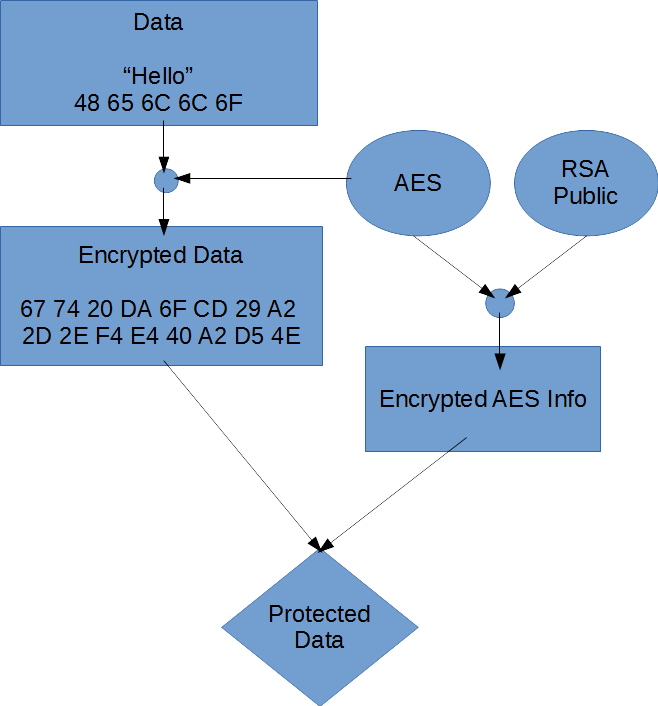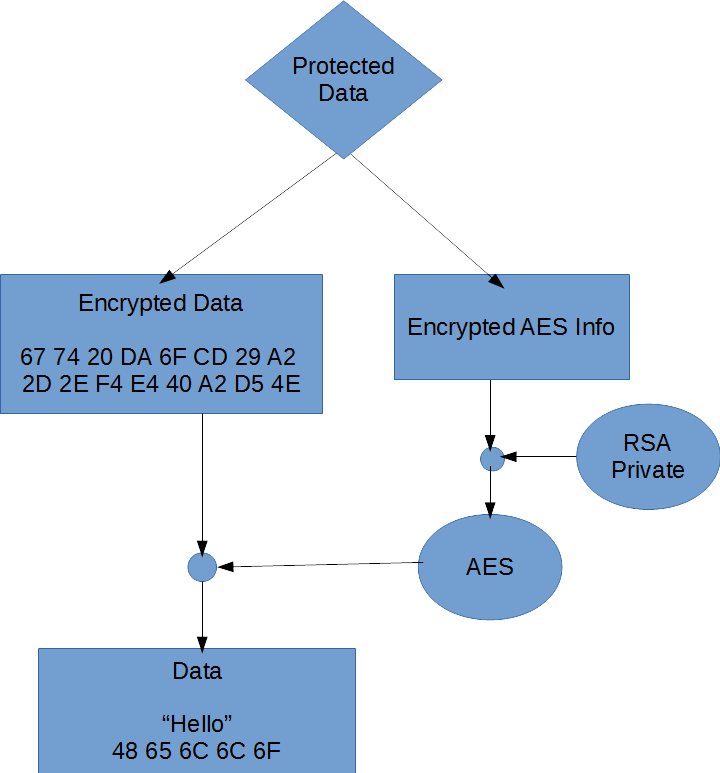# Asymmetric Cryptography in Practice

By | August 31, 2016

Encryption can be broken into either symmetric or asymmetric. Symmetric key cryptography is where the same cryptographic key is used for encryption and decryption. Asymmetric key (public-key) cryptography is where one key (the public key) is used for encryption and another key (the private key) is used for decryption. With the sort of added power that asymmetric (public-key) cryptography provides, one might think that asymmetric systems should always be used and that all symmetric algorithms should just be a relic of the past. However, asymmetric algorithms are limited by the size of the key used. For example, if RSA is used and the key length is 2048 bits then only data less than 2048 bits can be encrypted (the exact amount depends on the padding scheme used). Now one might think that asymmetric systems have no practical use, but they do in the realm of hybrid cryptosystems.

Hybrid cryptosystems are where the data is encrypted using a symmetric key and the symmetric key itself is encrypted using an asymmetric public key. In order to view the symmetrically encrypted data the symmetric key must be decrypted using the asymmetric private key. Then the symmetric key can be utilized to decrypt the data. TLS and PGP are examples of hybrid cryptosystems which take this approach.

So lets see some code of this concept in action. For this demo, I will utilize the cryptography support built into the .NET Framework. The demo will be written as a quick console application run through. In a real world application you would not be encrypting and decrypting the same message in memory. In the real world you would persist the key and data information in some serialized form such as XML and then transmit this information (likely over a network).

The encryption method:

Diagram:Code:

```private static byte[] EncryptMessage(byte[] dataBytes,
out byte[] encryptedAesKey, out byte[] encryptedAesIV, out byte[] rsaKeyBlob)
{
byte[] encryptedDataBytes;
using (var rsa = new RSACryptoServiceProvider())
{
Console.WriteLine("RSA Key Size: {0} bits", rsa.KeySize);
using (var aes = new AesCryptoServiceProvider())
{
Console.WriteLine("AES KEY: {0}", BitConverter.ToString(aes.Key));
Console.WriteLine("AES IV: {0}", BitConverter.ToString(aes.IV));
using (ICryptoTransform symEncryptor = aes.CreateEncryptor())
{
using (var outStream = new MemoryStream())
{
using (var cryptoStream = new CryptoStream(outStream,
symEncryptor, CryptoStreamMode.Write))
{
using (var inStream = new MemoryStream(dataBytes))
{
inStream.CopyTo(cryptoStream);
}
}

encryptedDataBytes = outStream.ToArray();
Console.WriteLine("Encrypted Data Bytes: {0}",
BitConverter.ToString(encryptedDataBytes));
}
}

rsaKeyBlob = rsa.ExportCspBlob(true);
encryptedAesKey = rsa.Encrypt(aes.Key, true);
encryptedAesIV = rsa.Encrypt(aes.IV, true);
Console.WriteLine("Encrypted AES KEY: {0}",
BitConverter.ToString(encryptedAesKey));
Console.WriteLine("Encrypted AES IV: {0}",
BitConverter.ToString(encryptedAesIV));
}
}

return encryptedDataBytes;
}
```

As you can see above, the data is encrypted using AES (AesCryptoServiceProvider) which is a symmetric encryption algorithm. Then the AES information (key and initialization vector) is then encrypted using RSA (RSACryptoServiceProvider) which is an asymmetric encryption algorithm. The term initialization vector was dropped in here and may be unfamiliar to some readers. For AES using cipher-block chaining (CBC) mode, the initialization vector (also referred to as a nonce) is a unique, random value which is added to the first block of data before encryption. Its use ensures that the same encrypted value does not imply the same encryption key. Without the use of an initialization vector there are several attacks which are then possible such as a chosen-plaintext attack.

The decryption method:

Diagram:Code:

```private static byte[] DecryptMessage(byte[] encryptedDataBytes,
byte[] encryptedKey, byte[] encryptedIv, byte[] keyBlob)
{
byte[] decryptedDataBytes;
using (var rsa = new RSACryptoServiceProvider())
{
rsa.ImportCspBlob(keyBlob);
byte[] aesKey = rsa.Decrypt(encryptedKey, true);
byte[] aesIV = rsa.Decrypt(encryptedIv, true);
Console.WriteLine("Decrypted AES KEY: {0}", BitConverter.ToString(aesKey));
Console.WriteLine("Decrypted AES IV: {0}", BitConverter.ToString(aesIV));
using (var aes = new AesCryptoServiceProvider())
{
using (ICryptoTransform symDecryptor = aes.CreateDecryptor(aesKey, aesIV))
{
using (var outStream = new MemoryStream())
{
using (var inStream = new MemoryStream(encryptedDataBytes))
{
using (var cryptoStream = new CryptoStream(inStream,
{
cryptoStream.CopyTo(outStream);
}
}

decryptedDataBytes = outStream.ToArray();
Console.WriteLine("Decrypted Data Bytes: {0}",
BitConverter.ToString(decryptedDataBytes));
}
}
}
}

return decryptedDataBytes;
}
```

First, the AES information (key and initialization vector) is decrypted using the RSA private key. Next, AES is used to decrypt the data portion.

Besides the key size being the limiting factor of what RSA can be used to encrypt – There is another bonus to using the hybrid approach… RSA and asymmetric algorithms in general are slow. AES on the other hand is relatively quick.

I hope that this demonstration helps a potential reader understand how to utilize asymmetric encryption in practice. As a parting note, I am no cryptographer or cryptography expert and the code here comes with no warranties. I am just an enthusiast and enjoy writing about the topic.

Source code can be downloaded here and is in the public domain.

This site uses Akismet to reduce spam. Learn how your comment data is processed.